# LinkedList in Java

In this article, we will be discussing the LinkedList in Java and its different operations like insertion, traversal.

Prerequisite: Linked List Introduction

As we know in the linked list we get a reference to the first node which is known as head and this first node has reference to the second node and so on … up to the last node, but the last node contains null in its reference indicating this is the end of the linked list as shown below: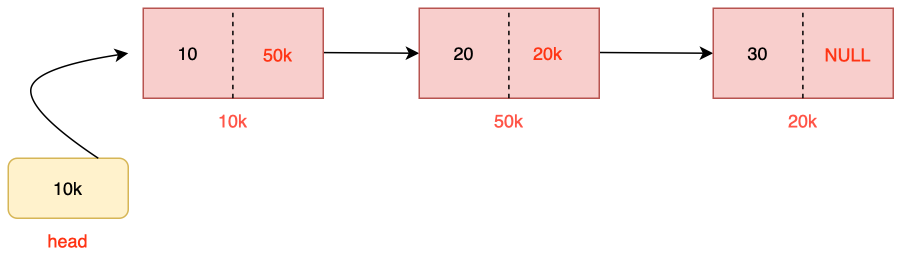Structure of node of linked list

These three nodes which have been created above will be getting memory in heap as in java anything which is created dynamically gets stored in heap. As the linked list is a nonprimitive data type that’s why it gets stored in heap.

Now you may ask why a linked list is a primitive data type?

And my answer will see the above diagram a node in a linked list contains the 2 things or two different types of data (data and address) which cannot be made through existing data types (like int, float, string)  so we will use a group of data types to create out new datatype as a node as shown below code.

``` static class Node {
int val;
Node next;
Node(int d)
{
val = d;
next = null;
}
}
```

In the above class, we have created two variables (val and node) and a constructor.

Variable with name val which will be going to contain data of linked list which will be deciding what the type of linked list means. If we have decided the type of val is a string then the type of linked list will be string, if int then it will be integer etc.

The second thing is quite interesting, which is storing the address of the self type means node here. So here we will be storing the address of the next node and null if the current node is the last node.

The third thing present here is the constructor which will be going to assign the value to the val and next variables. Assigning value to the next is optional here because in java if no value is assigned to the member then it by default becomes null.

Now let’s see how we can create the previously mentioned linked list:

We will be going to write the following code to create a linked list:

Code:

## Java Code

``````public class Main {
static class Node {
int data;
Node next;
Node(int d)
{
data = d;
next = null;
}
}
public static void main(String args[]) {
Node head = new Node(10);
Node temp1 = new Node(20);
Node temp2 = new Node(30);

temp1.next = temp2;
}
}
``````

When this line Node head = new Node(10); will get executed at that time the constructor will be called with data value 10 and this value assigned to the variable data and a new node will be created as shown below: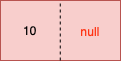Similarly for temp1, temp2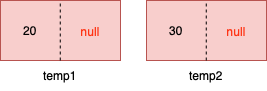When head.next = temp1;

Above line of called at that time the following link will get created: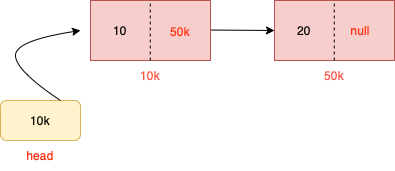And finally, the following linked list will be created:Now inside heap initially memory allocation will be going to be seen in the following fashion:Now after following 2 lines of code (after linking):

``` head.next = temp1;
temp1.next = temp2;
```

The memory address will be going to be looked like followsThe memory can or cannot be continuous here that’s why we have shown an unordered way of memory allocation.

Another method of creating a linked list can be shown below:

```public static void main(String args[]) {
Node head = new Node(10);
head.next = new Node(20);
head.next.next = new Node(30);
}
```

But the above-mentioned ways are not the best way or generalize for adding the elements in the linked list so now let’s see the different ways of inserting them in the linked list. For adding in the linked list we are provided with 3 ways:

a)Insert at the beginning/head.

b)Insert at the end.

c)Insert at any position.

Traversing  the linked list

Before going any further let’s see how we can display the linked list so that the above-mentioned operation could be seen in the output screen.

The following line of code will be going to be seen for displaying the linked list:

## Java Code

``````public static void printLinkedList(Node head){
Node curr = head;
while(curr != null){
System.out.print(curr.data+"->");
curr = curr.next;
}
}
``````

n the above code what we have done is we are traveling over the linked list and print the data associated with the node and moving ahead until null occurs. let’s take an example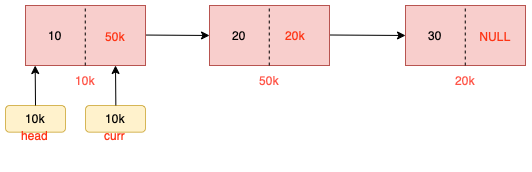When curr is at 10k address at that time the data associated will going to be printed as

` System.out.print(curr.data+"->"); `

This line of code has been written now with this line curr = curr. next; the curr pointer will going to be shifted one step ahead.

The new diagram will be going to look like this: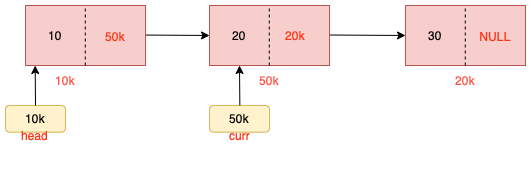And data 20 will be printed and this will continue to print

while(curr != null) this condition meets.

So we will get our output as 10->20->30.

Now we have seen how we can traverse and display the linked list so now it’s time to perform different operations in the linked list.

1)Insert at the Beginning/head:

Examples:

```Example 1:
Input: 10->20->30->null ,  insert 100
Output: 100->10->20-30->null

Example 2:
Input: null ,  insert 100
Output: 100->null```

Intuition:

In this method, a new node is always added at the front means every time a new node is added it becomes the new head of the linked list as seen in the examples stated above. So now let’s create a function named as insertAtHead() which takes 2 parameters as data and head. Where the head is the pointer pointing to the current head of the linked list and data is the value that we will be inserted at the beginning of the linked list.

Approach:

Let’s assume we didn’t have any node in the linked list so the head is pointing to null initially.

Coming to the line  Node temp = new Node(data);  this line will be creating the new node as shown,Now the initial value of the head is null therefore temp.next = head; when this line of code executes then we get null in address storing part of the linked list and we are returning the address of the head like a current node which is temp.

Now in the second scenario when we already have the values in the linked list at that time we will be having something like belowHere the head of the linked list is going to be pointing to this single node and we want that a node with data 100 should be added to it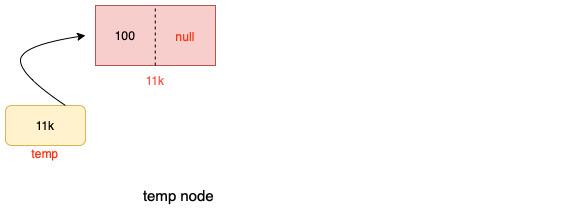This line of code helps in creating a new link between the previously created link list and the currently created node.  temp.next = head;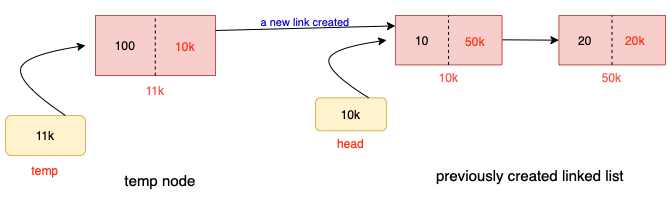And as soon as we return the new head as temp our linked list will be going to look like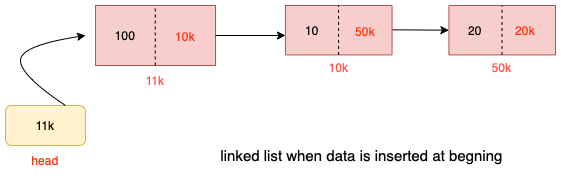Code:

## Java Code

``````public static Node insertAtHead(Node head, int data) {
Node temp = new Node(data);
{
}
else{
}

}``````

Time Complexity: O(1)

2) Insert at the end:

Examples:

```Example 1:
Input: 10->20->30->null ,  insert 100
Output: 10->20->30->100->null

Example 2:
Input: null ,  insert 100
Output: 100->null
```

Intuition:

While inserting a node at the end of the linked list we always search for the node having null at the end and add a new node after that node if there is no node present in the linked list at that time we treat the first node as the last node

Let’s create a function named insertAtHead() which takes 2 parameters as data and head. Where the head is the pointer pointing to the current head from where we have to start the traversal to find the node having null in its next and the second parameter is the data.

Approach:

Now let’s see understand the code line by line

Node temp = new Node(data);  as we know this line is going to create a new node as shownComing to the line   Node curr = head;  initially, we are making a new node pointing to the current head so that we could traverse the linked list to find the last nodeNow we will be going to traverse the linked list until we get fulfill our condition of while loop

while (curr.next != null). You will be wondering why we haven’t taken the condition of curr!=null this is because after this while loop we want that we should have a node so if the condition is curr!=null then we will not be able to get the node where we want to store the address of the newly created node.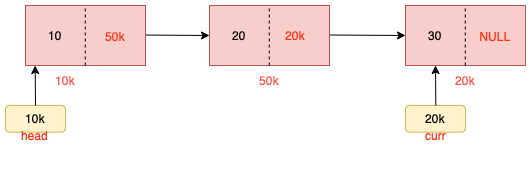Now as we get the access of the last node now our last work is to give the address of the newly created node to the current last node is this is done by this line of code curr.next = temp;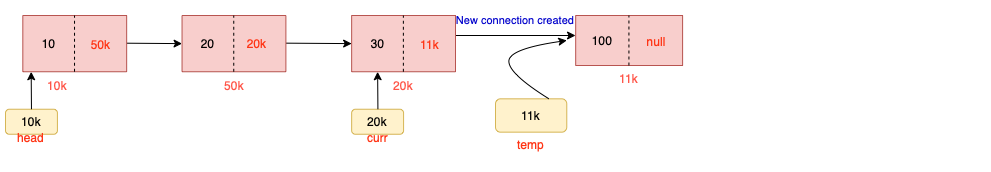So that’s how this new connection was created.

Code:

## Java Code

``````public class Main {
static class Node {
int data;
Node next;
Node(int d) {
data = d;
next = null;
}
}
public static void printll(Node head) {
while (head != null) {
}
System.out.println("null");
}
public static Node insertAtEnd(Node head, int data) {
Node temp = new Node(data);
if (head == null) {
return temp;
}
Node curr = head;
while (curr.next != null)
curr = curr.next;

curr.next = temp;
}
public static void main(String args[]) {
Node head = null;
}
}
``````

Output:

10->20->30->null

Time Complexity: O(n)

3)Insert at any position:

Examples:

```Example 1:
Input: 10->30->40->null ,  insert 100 , pos = 2
Output: 10->20->30->40null

Example 2:
Input: null ,  insert 100 , pos = 1
Output: 100->null

Example 3:
Input: 10->30->40->null ,  insert 100 , pos = 5
Output: 10->30->40null (no changes if pos is greater than size of linked list)
```

Intuition:

When we insert the node at any position we use the logic that until our count of position becomes 1 we traverse the linked list and at that position, we insert the data or node into it. Now let’s make a function that accepts the 3 parameters as head, data, and pos we all know the work of pos the thing which is new to us is pos, so pos here helps us to know in which position we will be adding the element.

Approach: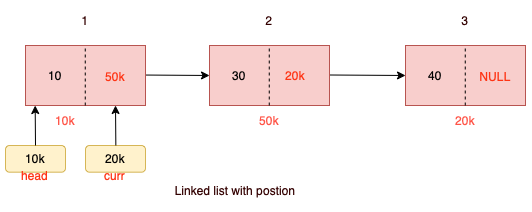Let’s take an example where we will be adding an element at pos 2 and the data be 20.

As we know the first line of code will be going to create a new node, after that we have an if condition telling about which is dealing with the condition if pos is one, there may be doubt in your mind that it is possible to call the insertAtBeg()  when pos is 1 so here you can definitely use that function and similarly if pos == length of linked list at that time you can use the function of insertAtEnd(), so now discuss the remaining code.

So coming to the for loop if we want to insert the element at the 2 pos then we will be checking for the i == pos-2 this is because we have to insert at pos == 1 not pos == 0 and as soon as we meet the condition we will set the next of curr pointer to address of the curr.next

And lastly, the address of the newly created will be containing the address of the curr node, and curr address will contain the address of the temp.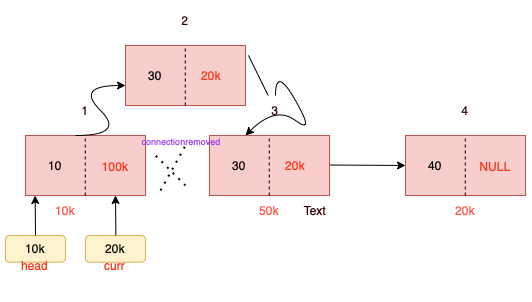And if the condition of position is more than the size of the linked list encountered it is going to be handled by curr != null condition as the current will come first as that of pos because we will reach the end if curr == null.

Code:

## Java Code

`````` public static Node insertAtPos(Node head, int data, int pos) {
Node temp = new Node(data);
if (pos == 1) {
return temp;
}
Node curr = head;
for (int i = 1; i <= pos - 2 && curr != null; i++) {
curr = curr.next;
}
if (curr == null) {